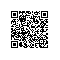# 二维数组作为函数参数传递剖析(C语言)(6.19更新第5种)

很多文章不外乎告诉你下面这几种标准的形式，你如果按照它们来用，准没错：//对于一个2行13列int元素的二维数组
//函数f的形参形式
f(int daytab) {...}

//以下两种可以忽略行数
f(int daytab[]) {...}

f(int (*daytab)) {...}甚至会有人告诉你多维数组作为参数传递可以省略第一维，其他维不能省略。然而你对这种形式并不满意：如果事先限定了二维数组的大小，函数的泛用性就要大打折扣了。因为你真正需要的，是可以处理事先未知行数和列数的二维数组的函数。当然也有文章提到类似下面的动态分配的方式，但作为函数参数传递有时不能成功，令人疑惑。

int **array;//array[M][N]
array = (int **)malloc(M *sizeof(int *));
for(i=0;i<M;i++)
array[i] = (int *)malloc(N *sizeof(int));

本文目的是深入剖析各个形式的二维数组，以及为了进行参数传递，如何写函数的形参表。更高维的数组可以做类似的推广。

下面先进行分析，文中讨论的地址空间是虚拟地址空间，是程序员看到的地址空间，不是实际的物理地址空间。

1.基本形式：二维数组在栈上分配，各行地址空间连续，函数参数使用文首提到的3种形式

最初接触二维数组时，可能只是在main()或某个函数里进行声明，然后直接使用：...
int array[M][N];
　　//array[][N] ={{...},...,{...}}; is ok
//array[][] = {{...},...,{...}}; is wrong

   ...
//使用array[m][n]这种分配是在栈上进行的，能够保证所有元素的地址空间连续。这样，array[i][j] 和 *(*(array +i) +j)是一样的，程序是知道array+i的i实际上偏移了i*N个单位，这也导致了在二维数组array中，使用下标array和array是访问的同一个元素，尽管后者的下标对于一个3*3矩阵来说是非法的，但这并不影响访问。

这种形式，无论是数组定义还是函数都不够泛用，两个维度在编译前就定好了，唯一可以做的就是把维度M、N声明为宏或者枚举类型，但这仍不能避免每次修改后都要重新编译。

2.数组传参形式：二维数组在栈上分配，各行地址空间连续，函数参数使用指针形式

当把这种二维数组的指针直接作为参数传递时，数组名退化为指针，函数并不知道数组的列数，N对它来说是不可见的，即使使用*(*(array +i) +j)，第一层解引用失败。这时，编译器会报warning，运行生成的文件会发生segment fault。那么，为了指导这个函数如何解引用，也就是人为地解引用，需要把这个二维数组的首元素地址传给函数，于是就变成了下面的形式：#include <stdio.h>
#include <stdlib.h>
#include <assert.h>
int func(int *array, int m, int n) {
int i,j;
for(i=0;i<m;i++) {
for(j=0;j<n;j++)
printf("\t%d", *(array +i*n +j));
printf("\n");
}
return 0;
}

int main(int argc,char** argv) {
int m=3,n=3,i;
int array[] = {{1,2,3},{4,5,6},{7,8,9}};
func(*array,m,n);
return 0;
}也可以写成int fun(int *array,int m,int n) {
int i,j;
for(i=0;i<m;i++)
for(j=0;j<n;j++)
printf("%d ", *((int*)array + n*i + j));
return 0;
}
int main() {
int array = {
{1,2,3},
{4,5,6},
{7,8,9}
};
fun((int *)array,3,3);
return 0;
}但是意图没有上一种清晰，并不推荐。

你可能会问，为什么下面的不行？原因其实和上面提到的一样，第一次解引用时，函数并不知道数组的列数，从而导致失败。准确的说，是因为数组实际类型是int ，在作为右值时可以被转化为int (*)，它们都和int **不同，自然不可用。（感谢garbageMan在回复中指出）int func(int **array, int m, int n) {
...
printf("\t%d", *(*array +i*n +j));
...
}

int main() {
int array = {
{1,2,3},
{4,5,6},
{7,8,9}
};
...
func(array,3,3);
...
}3.动态数组形式：二维数组在堆上分配，各行地址空间不一定连续，函数参数使用指针形式

第2种虽然函数参数的限定降低了，但仍需要在栈上预先分配一定大小的二维数组，程序整体并不是完全的泛用。为了进一步提高泛用性，把二维数组空间的分配也动态化，使用malloc()在堆上分配空间，重复一下前言中的方式如下：

int **array;
array = (int **)malloc(m *sizeof(int *));
for(i=0;i<M;i++)
array[i] = (int *)malloc(n *sizeof(int));

这时，在分配空间的作用域里，对0<=i<M,0<=j<N，array[i][j]的访问完全没有问题。那么，对应地，函数写作

int func(int **array,int m,int n) {
...
printf("%d ", *(*(array+i)+j));
...
}

值得注意的是，虽然malloc()每次分配的空间在地址上是连续的，但是多次malloc()分配的空间之间并不一定是连续的，这与在栈上分配的二维矩阵有着根本的不同，对于二维数组array，不能再用array来访问array了，前者地址越界。

4.折中形式：用堆上分配的一维数组表示二维数组，函数参数使用指针形式

用一维数组来实现二维数组，是一种折中方案，但是很好理解，也不易出错。这样分配的数组空间是连续的。使用时需要把两维下标转化为一维下标。#include <stdio.h>
#include <stdlib.h>
#include <assert.h>
int func(int *array, int m, int n) {
int i,j;
for(i=0;i<m;i++) {
for(j=0;j<n;j++)
printf("\t%d",*(array+i*n+j));
printf("\n");
}
return 0;
}

int main(int argc,char** argv) {
int m,n,i;
int *array;
assert(argc == 3);
m = atoi(argv);
n = atoi(argv);
array = (int*)malloc(m*n*sizeof(int));
for(i=0;i<m*n;i++)
array[i] = i;
func(array,m,n);
return 0;
}5.较新的编译器：用栈上分配的直到执行时才确定大小的二维数组

C90不支持这种形式，C99支持，因此一些较新的编译器可以对下面的代码进行执行。注意print()的参数顺序不能改变。void print(int x, int y, int a[x][y]){
printf("\n");
int i, j;
for(i = 0; i < x; i++){
for(j = 0; j < y; j++)
printf("%d     ", a[i][j]);
printf("\n");
}
}

// Function to initialize the two-dimensional array
void init_2d(int *a, int x, int y){
int i, j;
for(i = 0; i < x; i++){
for(j = 0; j < y; j++){
a[i*y + j] = i + j;
}
printf("\n");
}
}

int main(){
int m , n ;
scanf("%d %d",&m,&n);
int a[m][n];  // a two dimensional whose size has been defined using variables
init_2d(a, m, n);
print(m, n, a);
}（2013.7.28更新）

另外，这种分配方式仍然是在栈上，相关讨论可见于http://bbs.csdn.net/topics/90350681

• 其实所谓的二维数组，在K&R上只是指预先分配好大小的形如int a[M][M]这样的数组，它存在于栈上；而实际使用的在堆空间利用malloc动态分配空间的并不是这种，只是用的人多了，把后者叫成二维数组了（我不认为把后者也称为二维数组是标准的说法）。再加上我们经常用它来处理矩阵，“标准的”二维数组、“动态的”“二维数组”、矩阵这三个概念就混在了一起。矩阵是可以用这两种二维数组表示的，而对于这两种不同的二维数组，函数传参的方式也不完全相同，不能随意混用。
• C99对于多维数组的描述：

If E is an n -dimensional array ( n ≥ 2) with dimensions i × j × ... × k , then E (used as other than an lvalue) is converted to a pointer to an ( n − 1)-dimensional array with dimensions j × ... × k . If the unary * operator is applied to this pointer explicitly, or implicitly as a result of subscripting, the result is the pointed-to ( n − 1)-dimensional array which itself is converted into a pointer if used as other than an lvalue. It follows from this
that arrays are stored in row-major order (last subscript varies fastest).

• 栈上分配的二维数组数组名int array的真实类型是int [ ][ ]，在作为右值时才被转化为(int *array)[N] （感谢 garbageMan指出），和int **是不同的。把前者进行强制转换为后者，在函数中对元素操作也会导致段错误，下面用图来说明二者区别：使用钉钉扫一扫加入圈子
+ 订阅